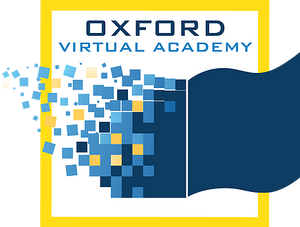# Math 6

This course will build a solid foundation in mathematics by exploring topics such as decimals, fractions, expressions, equations, graphing, measurement, and statistics. This course will introduce students to ratios, percents, and geometry and will also teach students how to collect and interpret data and display their findings through graphs. Students will learn to recognize patterns and how to work with variables. Prime numbers, factoring, and divisibility rules will be covered as well, and students will work with adding, subtracting, multiplying, and dividing fractions. This course includes discussions of ratios and solving proportions. Geometry concepts such as triangles, angels, perimeter, and area will also be covered. As the course progresses, students will learn about circles, 3-D figures, and finding surface area and volume of different prisms. Finally, students will explore graphing and how integers are used in real-world situations.

* Prerequisites: Math 5